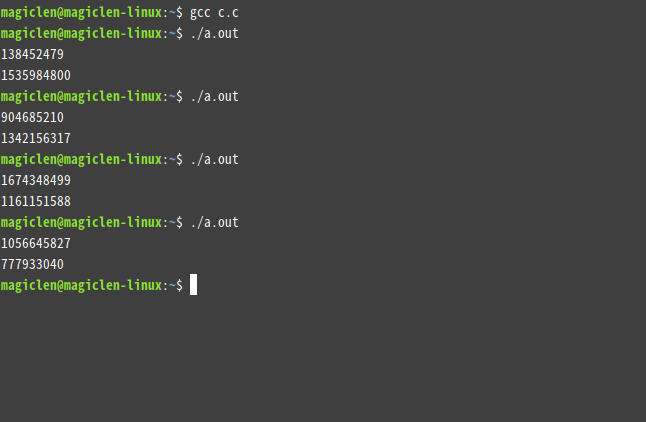```#include<stdio.h>
#include<stdlib.h>

int main() {
int rnd1 = rand();
int rnd2 = rand();

printf("%d\n", rnd1);
printf("%d\n", rnd2);
return 0;
}``````#include<stdio.h>
#include<stdlib.h>
#include<time.h>

int main() {
srand(time(NULL));

int rnd1 = rand();
int rnd2 = rand();

printf("%d\n", rnd1);
printf("%d\n", rnd2);
return 0;
}``````extern crate rand;

use rand::Rng;

fn main() {

println!("{}", rnd);
}```

```extern crate rand;

use rand::Rng;

fn main() {

println!("{}", rnd);
}```

```extern crate rand;

use rand::Rng;

fn main() {

println!("{}", rnd);
}```

### Random Number

「Random Number」是筆者開發的套件，能夠以便利的寫法來產生隨機數值。

Crates.io

https://crates.io/crates/random-number

Cargo.toml

random-number = "*"

#### 巨集的使用

##### random

`random`巨集的基本用法如下：

```#[macro_use]
extern crate random_number;

let n: u8 = random!();
println!("{}", n); // 0 ~ 255

let n: f64 = random!();
println!("{}", n); // 0.0 ~ 1.0

let n: u8 = random!(..=10);
println!("{}", n); // 0 ~ 10

let n: u8 = random!(..=9);
println!("{}", n); // 0 ~ 9

let n: u8 = random!(10..);
println!("{}", n); // 10 ~ 255

let n: i8 = random!(-2..=12);
println!("{}", n); // -2 ~ 12

let n: u8 = random!(12, 20);
println!("{}", n); // 12 ~ 20

let n: u8 = random!(20, 12);
println!("{}", n); // 12 ~ 20```

```#[macro_use]
extern crate random_number;

let n: u8 = random!(rng);
println!("{}", n); // 0 ~ 255

let n: u8 = random!(..=10, rng);
println!("{}", n); // 0 ~ 10

let n: u8 = random!(20, 12, rng);
println!("{}", n); // 12 ~ 20```
##### random_fill

`random_fill`巨集的語法和`random`巨集差不多，只不過要在第一個參數多傳一個可變的切片，這個巨集會用隨機數值填滿這個切片。

```#[macro_use]
extern crate random_number;

let mut a = [0i8; 32];
random_fill!(a, -2..=12);

println!("{:?}", a);```

#### `random_ranged`和`random_fill_ranged`函數

```#[macro_use]
extern crate random_number;

let var_range = 1..=10;

let n: u8 = random!(var_range); // compile error```

```extern crate random_number;

let var_range = 1..=10;

let n: u8 = random_number::random_ranged(var_range);

println!("{:?}", a);```